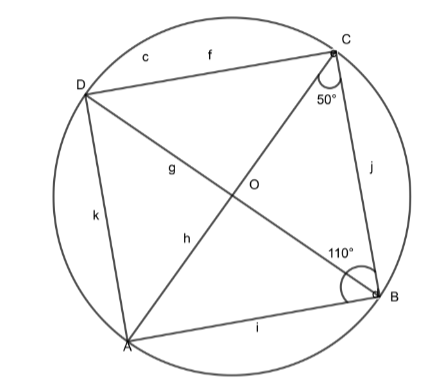Courses
Courses for Kids
Free study material
Free LIVE classes
MoreLIVE
Join Vedantu’s FREE Mastercalss

# ABCD is a cyclic quadrilateral whose diagonals intersect at O. If $\angle ACB=50{}^\circ$ and $\angle ABC=110{}^\circ$, find $\angle BDC$.Verified
360.6k+ views
Hint: We can solve this problem by using the property of angle inscribed by the arc, i. e. Angles inscribed by the same arc on the circumference of a circle are always EQUAL, and the property of a triangle.

Firstly, we will write the given values,
$\angle ACB=50{}^\circ$ and $\angle ABC=110{}^\circ$……………………………….. (1)
To find the required angle we should know the key concept which is given below,
Angles inscribed by the same arc on the circumference of a circle are always EQUAL, which means, angles made by arc BC at point D and A on the circumference of the circle are equal.
$\therefore \angle BDC=\angle BAC$……………………………………………….. (2)
To find the value of $\angle BAC$ consider$\vartriangle ABC$,

As the sum of angles of a triangle is always $180{}^\circ$
$\therefore \angle ABC+\angle BAC+\angle ACB=180{}^\circ$
Put the values of (1) in the above equation,
$\Rightarrow 110{}^\circ +\angle BAC+50{}^\circ =180{}^\circ$
$\Rightarrow \angle BAC=180{}^\circ -160{}^\circ$
$\Rightarrow \angle BAC=20{}^\circ$
Now put the value of equation (2) in the above equation,
$\Rightarrow \angle BDC=\angle BAC=20{}^\circ$
$\therefore \angle BDC=20{}^\circ$
Therefore the value of $\angle BDC$ is $20{}^\circ$.

Note: In the problem they have just mentioned that diagonals are passing through point O, but it is not clear that O is the centre of the circle. If we consider YOU as center then, $\angle DCA$ will become $40{}^\circ$ and we will get the final answer as $\angle BDC=50{}^\circ$ which is a wrong answer.
Last updated date: 19th Sep 2023
Total views: 360.6k
Views today: 5.60k